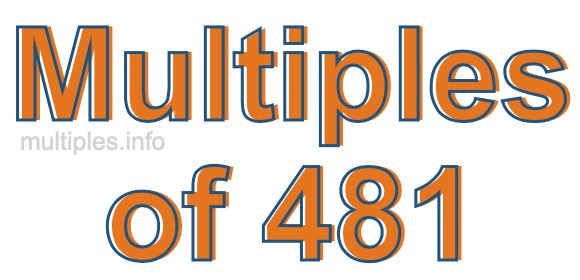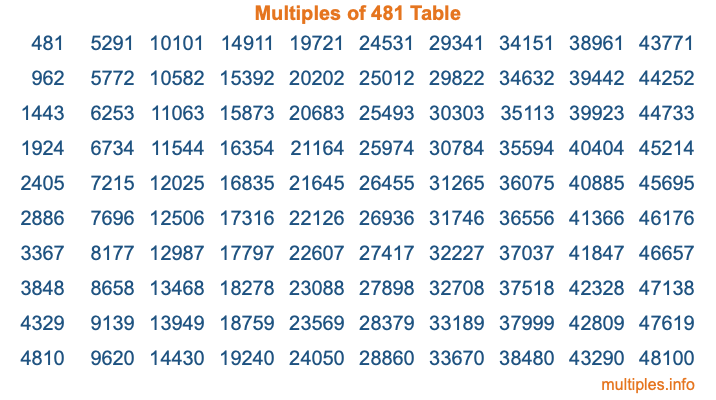Multiples of 481Welcome to the Multiples of 481 page. Here we will first teach you everything you will ever need to know about the multiples of 481, and then give you a study guide summary of everything we taught you to make sure you remember it all. Use this page to look up facts and learn information about the multiples of 481. This page will make you a multiples of four hundred eighty-one expert!

Definition of Multiples of 481
Multiples of 481 are all the numbers that when divided by 481 equal an integer. Each of the multiples of 481 are called a multiple. A multiple of 481 is created by multiplying 481 by an integer.

Therefore, to create a list of multiples of 481, you start with 1 multiplied by 481, then 2 multiplied by 481, then 3 multiplied by 481, and so on for as long as you want. Thus, the list of the first five multiples of 481 is 481, 962, 1443, 1924, and 2405. To see a larger list of multiples of 481, see the printable image of Multiples of 481 further down on this page. We also have a category where you can choose any nth multiple of 481.

Multiples of 481 Checker
The Multiples of 481 Checker below checks to see if any number of your choice is a multiple of 481. In other words, it checks to see if there is any number (integer) that when multiplied by 481 will equal your number. To do that, we divide your number by 481. If the the quotient is an integer, then your number is a multiple of 481.

Is  a multiple of 481?

Least Common Multiple of 481 and ...
A Least Common Multiple (LCM) is the lowest multiple that two or more numbers have in common. This is also called the smallest common multiple or lowest common multiple and is useful to know when you are adding our subtracting fractions. Enter one or more numbers below (481 is already entered) to find the LCM.

Check out our LCM Calculator if you need more details about the Least Common Multiple or if you need the LCM for different numbers for adding and subtraction fractions.

nth Multiple of 481
As we stated above, 481 is the first multiple of 481, 962 is the second multiple of 481, 1443 is the third multiple of 481, and so on. Enter a number below to find the nth multiple of 481.

th multiple of 481

Multiples of 481 vs Factors of 481
481 is a multiple of 481 and a factor of 481, but that is where the similarities end. All postive multiples of 481 are 481 or greater than 481. All positive factors of 481 are 481 or less than 481.

Below is the beginning list of multiples of 481 and the factors of 481 so you can compare:

Multiples of 481: 481, 962, 1443, 1924, 2405, etc.

Factors of 481: 1, 13, 37, 481

As you can see, the multiples of 481 are all the numbers that you can divide by 481 to get a whole number. The factors of 481, on the other hand, are all the whole numbers that you can multiply by another whole number to get 481.

It's also interesting to note that if a number (x) is a factor of 481, then 481 will also be a multiple of that number (x).

Multiples of 481 vs Divisors of 481
The divisors of 481 are all the integers that 481 can be divided by evenly. Below is a list of the divisors of 481.

Divisors of 481: 1, 13, 37, 481

The interesting thing to note here is that if you take any multiple of 481 and divide it by a divisor of 481, you will see that the quotient is an integer.

Multiples of 481 Table
Below is an image of the first 100 multiples of 481 in a table. The table is in chronological order, column by column. The first column has the first ten multiples of 481, the second column has the next ten multiples of 481, and so on.The Multiples of 481 Table is also referred to as the 481 Times Table or Times Table of 481. You are welcome to print out our table for your studies.

Negative Multiples of 481
Although not often discussed or needed in math, it is worth mentioning that you can make a list of negative multiples of 481 by multiplying 481 by -1, then by -2, then by -3, and so on, to get the following list of negative multiples of 481:

-481, -962, -1443, -1924, -2405, etc.

Multiples of 481 Summary
Below is a summary of important Multiples of 481 facts that we have discussed on this page. To retain the knowledge on this page, we recommend that you read through the summary and explain to yourself or a study partner why they hold true.

There are an infinite number of multiples of 481.

A multiple of 481 divided by 481 will equal a whole number.

481 divided by a factor of 481 equals a divisor of 481.

The nth multiple of 481 is n times 481.

The largest factor of 481 is equal to the first positive multiple of 481.

481 is a multiple of every factor of 481.

481 is a multiple of 481.

A multiple of 481 divided by a divisor of 481 equals an integer.

481 divided by a divisor of 481 equals a factor of 481.

Any integer times 481 will equal a multiple of 481.

Multiples of a Number
Here you can get the multiples of another number, all with the same attention to detail as we did for multiples of 481 on this page.

Multiples of
Multiples of 482
Did you find our page about multiples of four hundred eighty-one educational? Do you want more knowledge? Check out the multiples of the next number on our list!